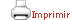Teaching plan for the course unit

 CloseGeneral information

Course unit name: Advanced Model Theory

Course unit code: 569075

Coordinator: Enrique Casanovas Ruiz Fornells

Department: Department of Mathematics and Computer Science

Credits: 5

Single program: S

 Estimated learning time Total number of hours 125

 Face-to-face and/or online activities 42
 -  Lecture with practical component Face-to-face 42
 Supervised project 18
 Independent learning 65

 Recommendations

 Further recommendations It is very recommendable to have followed with success  a basic model theory course.

 Competences to be gained during study

 To be able to carry out independent study of standard textbooks  and journal articles on model theory.   To understand the interest and potential impact of model theory and its applications to mathematics.   To be able to handle standard model-theoretic problems, formulating them with accuracy  and knowing the usual techniques to solve them.

 Learning objectives
 Referring to knowledge A deep knowledge of the basic notions, tools and results from model theory (saturation, definability, prime models, indiscernibles, etc). Introduction to the elementary notions of stability theory (Morley and Baldwin-Lachlan theorems) Familiarity with examples of complete first-order theories, particularly vector spaces and algebraically closed fields.  Referring to abilities, skills To be able to prove completeness, omega-categoricity and quantifier elimination for given examples of first-order theories. To characterize definable sets in models of a given theory. To classify the complexity of a theory from the point of view of stability theory

 Teaching blocks

1. PRELIMINARIES

*  Back and forth, saturation, the monster model, quantifier elimination, model-completeness,  and omega-categoricity.

2. DEFINABILITY

*
Definable, type-definable and invariant relations.  Beth and Svenonious theorems. Definable and algebraic closure.  Imaginaries.

3. PREGEOMETRIES

*
Closure operators with exchange. Independence, basis and dimension. Modularity. Vector spaces.

4. RANKS

*
Cantor-Bendixson rank. Morley rank.  Omega-stability and superstability. Strongly minimal sets. Algebraically closed fields.

5. PRIME MODELS

*  Omitting types. Prime and atomic models. Indiscernibles.  Two cardinal theorems.

6. CATEGORICITY

*
Morley and Baldwin-Lachlan’s theorems.

 Teaching methods and general organization

 Detailed exposition in class, with examples and full proofs. Asking questions and proposing problems to be solved by the student. Class discussions on the main notions, examples, statements and relevant proofs. Presentation of a topic by a student, previously discussed with the instructor.

 Official assessment of learning outcomes

 Weekly exercises. 40 % Oral presentation of a topic 10 %  Two partial exams  25 % each.   Examination-based assessment Final examination

Book

 These Lecture Notes will be delivered during the course.

E. Casanovas.  Teoría de Modelos (Lecture Notes).  1999.

 Available at http://www.ub.es/modeltheory/casanovas

C.C. Chang and H.J. Keisler.  Model Theory. North Holland PC,  3rd ed. 1990.

W. Hodges. Model Theory.  Cambridge UP 1993.

D. Lascar. Stability in Model Theory. Longman Sci. and Tech.  1987.

D. Lascar. La théorie des modèles en peu de maux. Cassini, Paris 2009.

D. Marker. Model Theory: an introduction. Springer 2002.

D. Marker, M. Messmer and A. Pillay. Introduction to the Model Theory of Fields.  Lecture Notes in Logic 5. Springer 1996.

A. Pillay. An introduction to Stability Theory.  Oxford UP  1983.

A. Pillay. Geometrical Stability Theory.  Oxford UP. 1996.

B. Poizat.  Cours de théorie des modèles. Offilib. Villeurbanne 1985.

K. Tent and M. Ziegler. A course in Model Theory.  Lecture Notes in Logic 40. Cambridge UP 2012.